iOS Application Security Part 29 - Insecure or Broken Cryptography

#### Posted by Prateek Gianchandani

In this article we will look at an example of Insecure or Broken Cryptography which is a common vulnerability found in most iOS applications. This vulnerability occurs when the data stored on the device is not encrypted properly thereby allowing a malicious user to gain access to that information. There could be many reasons for an improper implementaion of encrytption, using hardcoded keys for encryption, bad algorithms etc can all be the cause for an implementation that is not secure.

I would recommend you have a look at Apple’s documentation on Encrypting and hashing data.

In this article, we will look at an example of how we can spot and break an incorrectly implemented encrytion technique. For this article, we will be testing on the application InsecureCryptography-Demo that you can download from my Github profile. Download it and run on the simulator or on the device. Let’s look at what this application does. Once you start the application for the first time, it asks you to set up a new password to get started.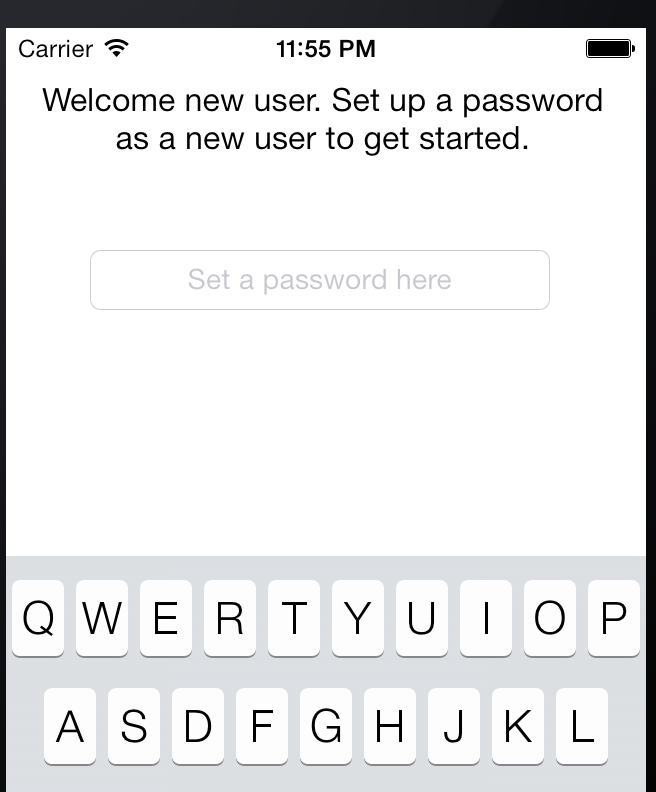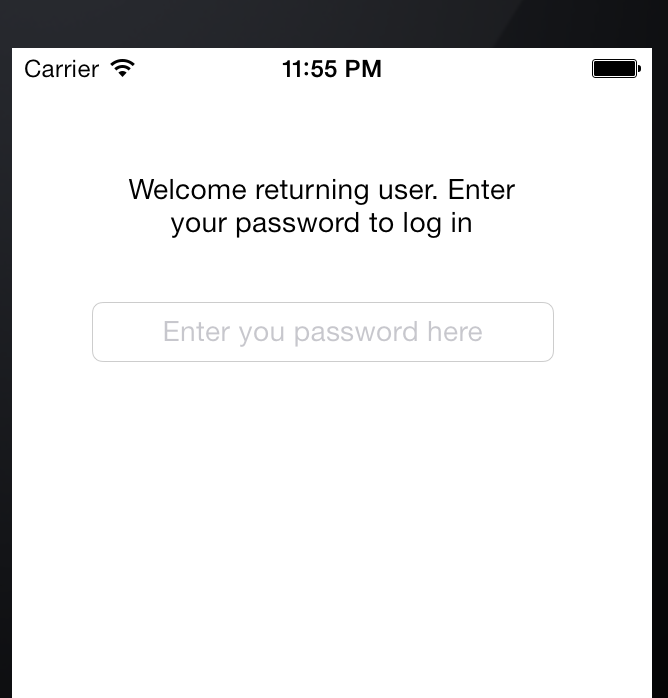So lets assume i open this application once the password has already been set. Our task is to bypass this login check. Some of the things that are clear are that this password is being stored locally on the device as no network activity was noted. You can use a proxy like Burpsuite to observe the traffic and see that there is no network traffic, so everything is being done locally.

Let’s try and open the application in Hopper. Please check article 28 in this series to know more about Hopper. Go to Hopper and go to File->Read Executable to Disassemble.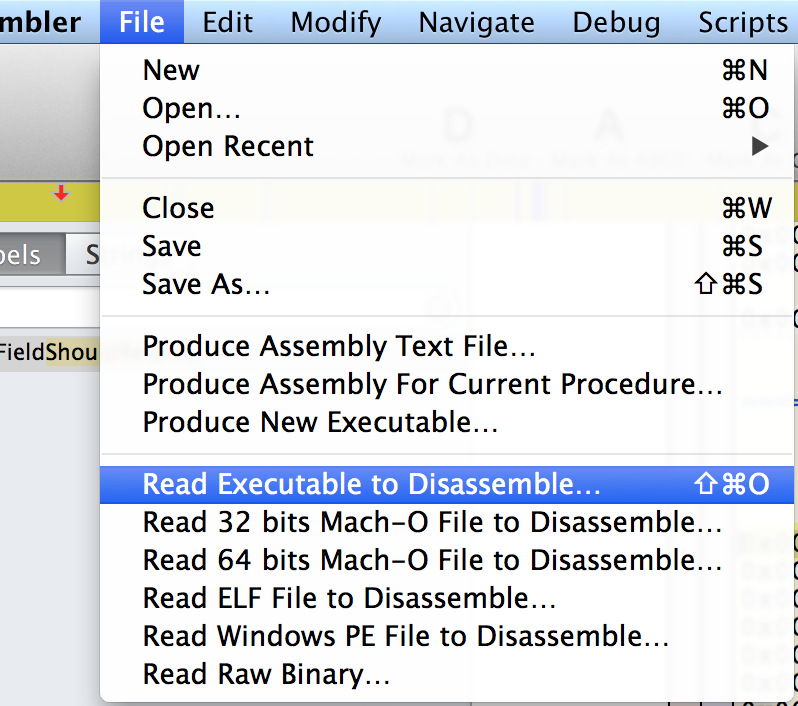If you have successfully run the application using Xcode, it will install the application in the iOS simulator. Now our task is to find the location of the application binary on our system so we can provide it to Hopper. If you run an application in Xcode, it will generate an application directory inside the folder /Users/\$username/Library/Application Support/iPhone Simulator/\$ios version of simulator/Applications/. In my case, the location is /Users/Prateek/Library/Application Support/iPhone Simulator 7.0.3/Applications/. Once you are in this directory, you have to find your application folder. Using the command ls -al will give you the created date of these folders. The latest one would be our application.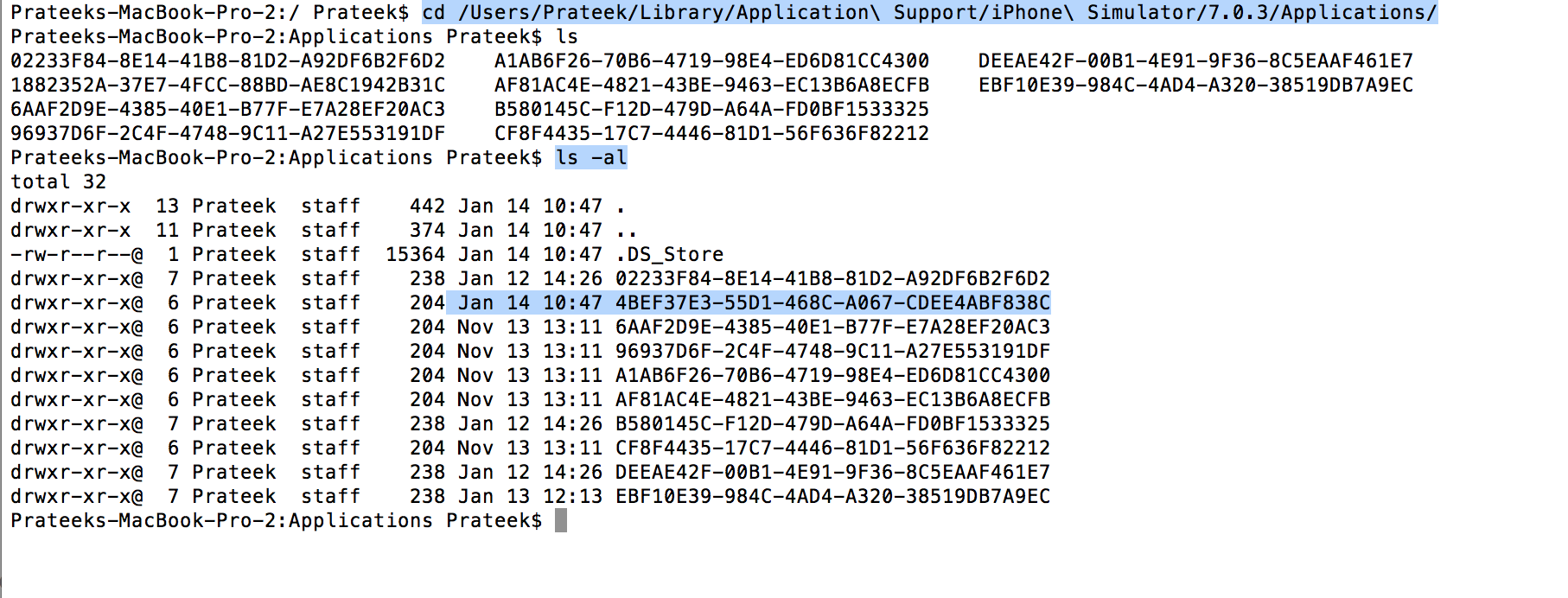Open this folder in Finder using the command open \$directoryName and right click on the .app package and click on Show Package Contents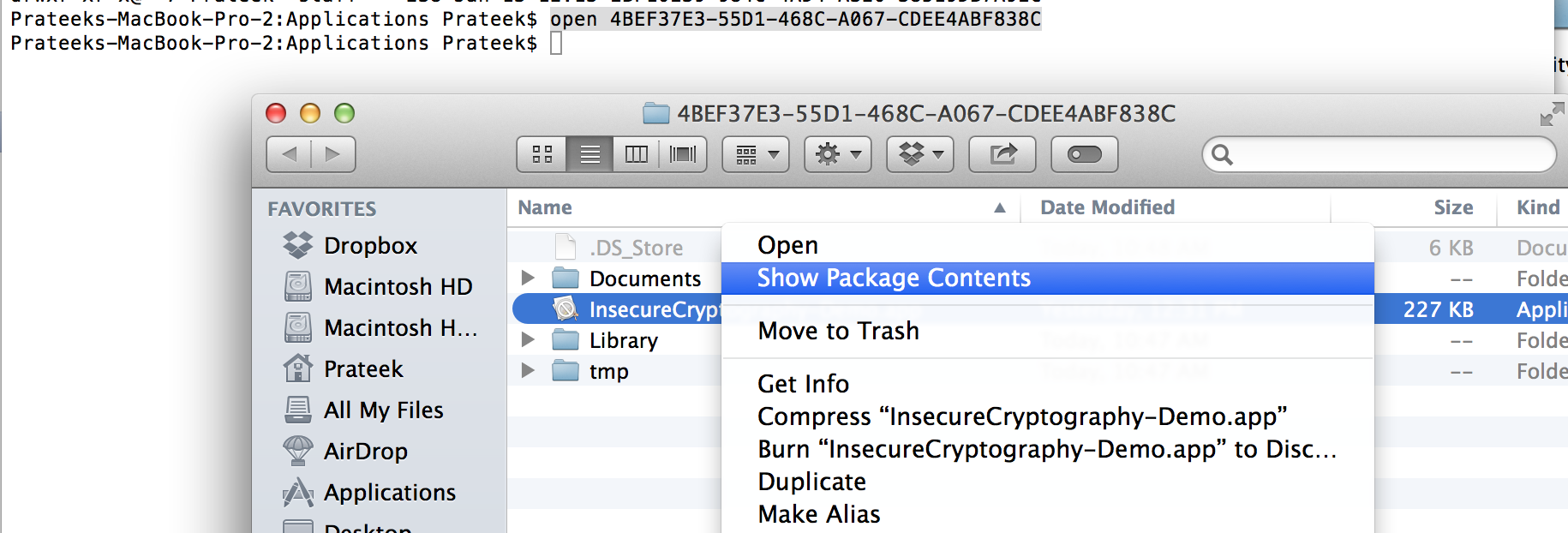Provide this executable to Hopper to disassemble.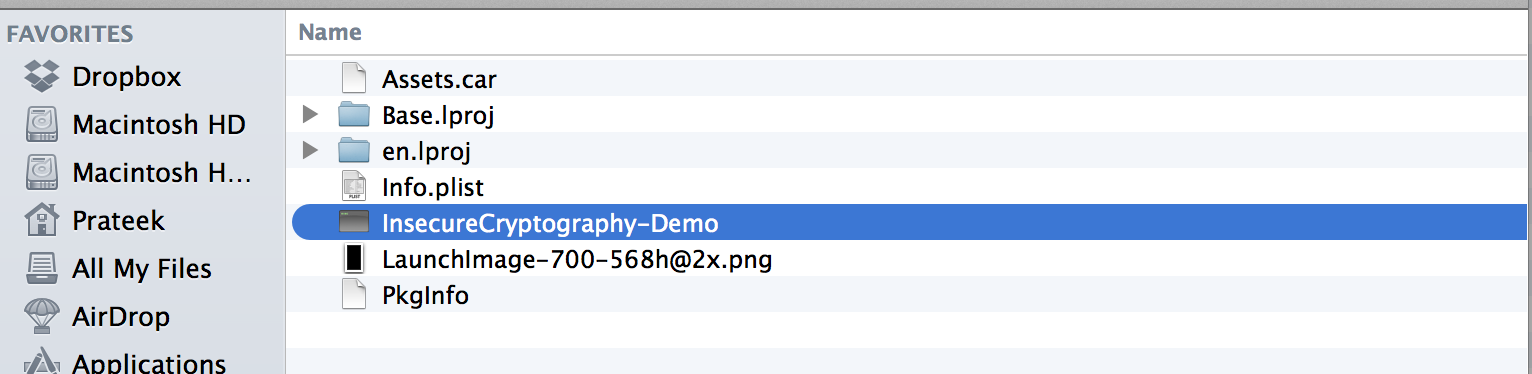Once Hopper has analyzed the executable and produced the dissassembly, here is what you get.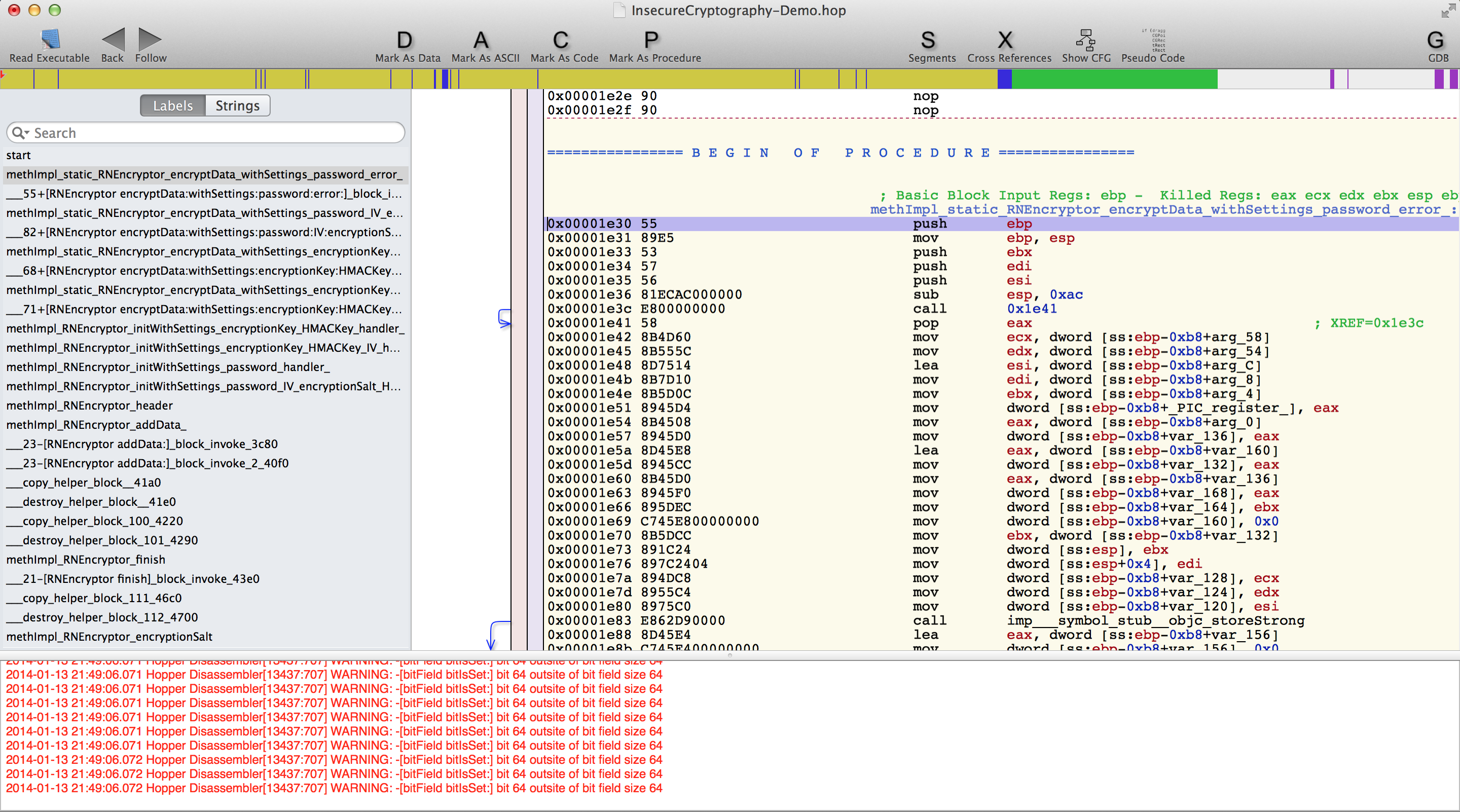On the left hand side, you can see stuff like RNEncryptor etc. Looks like this class is being used to encrypt data.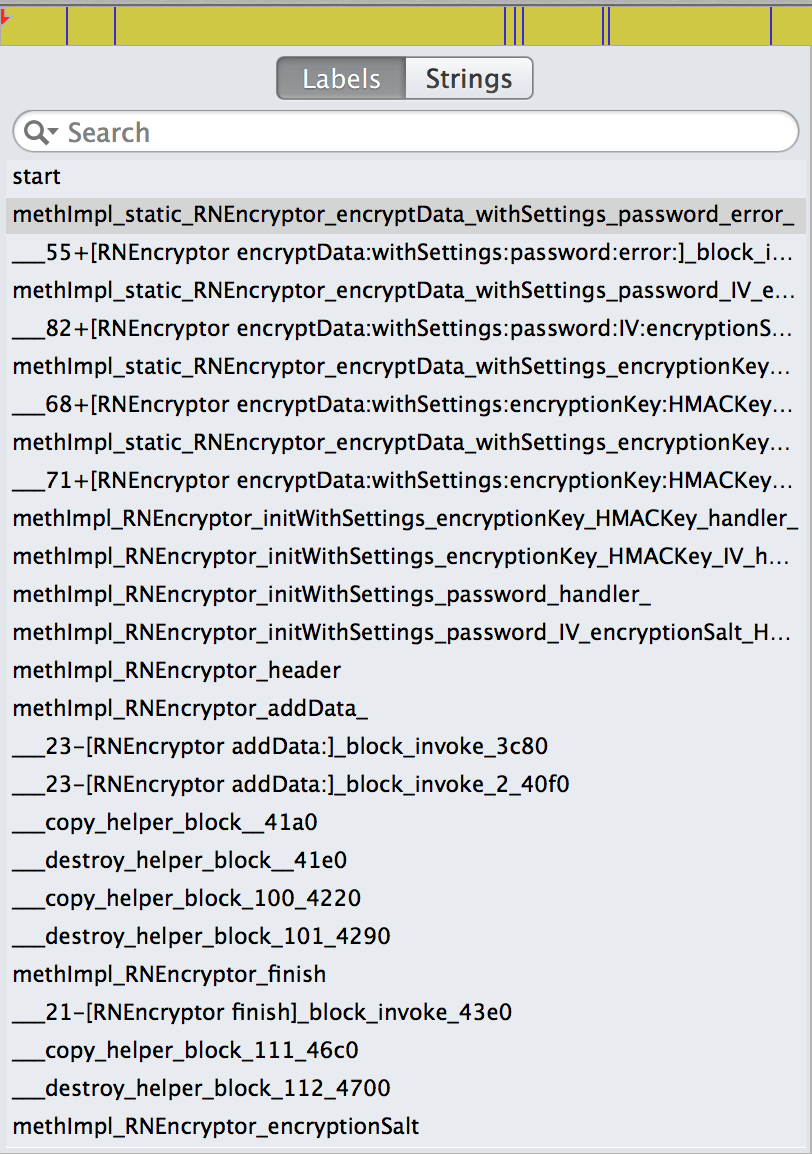On google searching RNEncryptor, we find that it is a open source Github class used for encrypting data. Anyways, our task is to bypass the login. So lets search for the method that gets called to perform the authentication check. Let’s go back to the app. If you look at the image below, there is no login button to tap. The login check happens once you tap on the return button in the text field.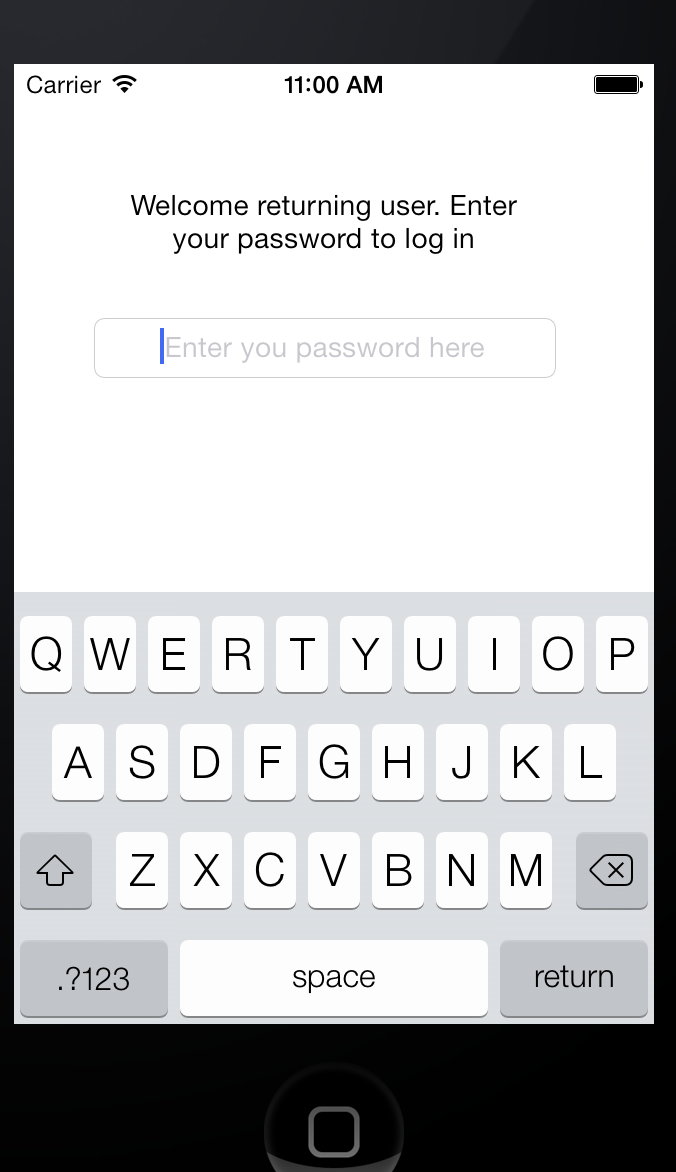And if you are a bit familiar with iOS development you should know that if the current view controller is a delegate of this text field, then the method that will be called is -(BOOL)textFieldShouldReturn:(UITextField *)textField. So lets search for this method in the labels section.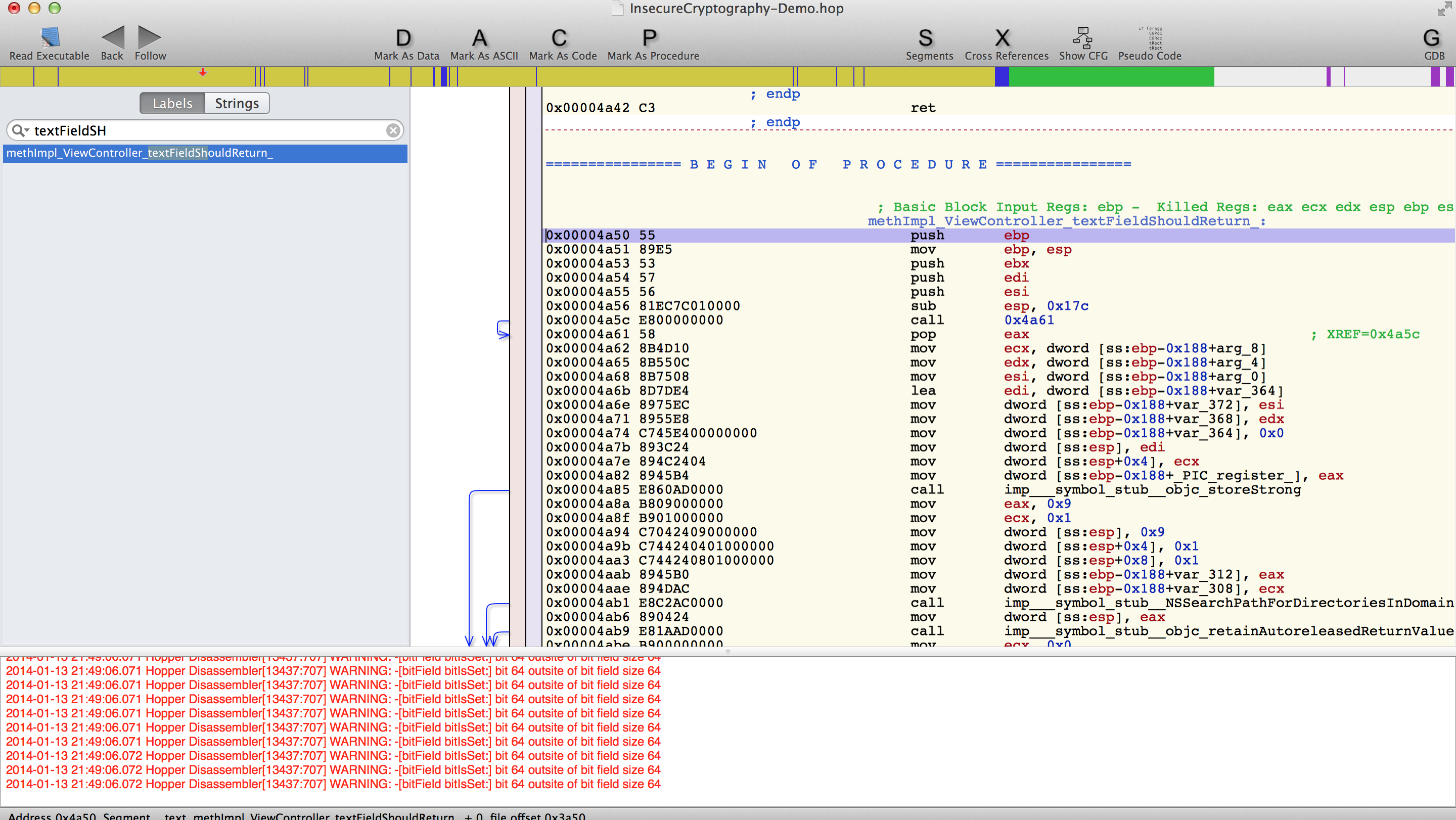Ok, we can see the disassembly. That’s pretty cool. But you know what’s more cool ? Checking out the pseudo code for this function. Let’s click on the Pseudo Code button on the top right to check out the Pseudo code for this function.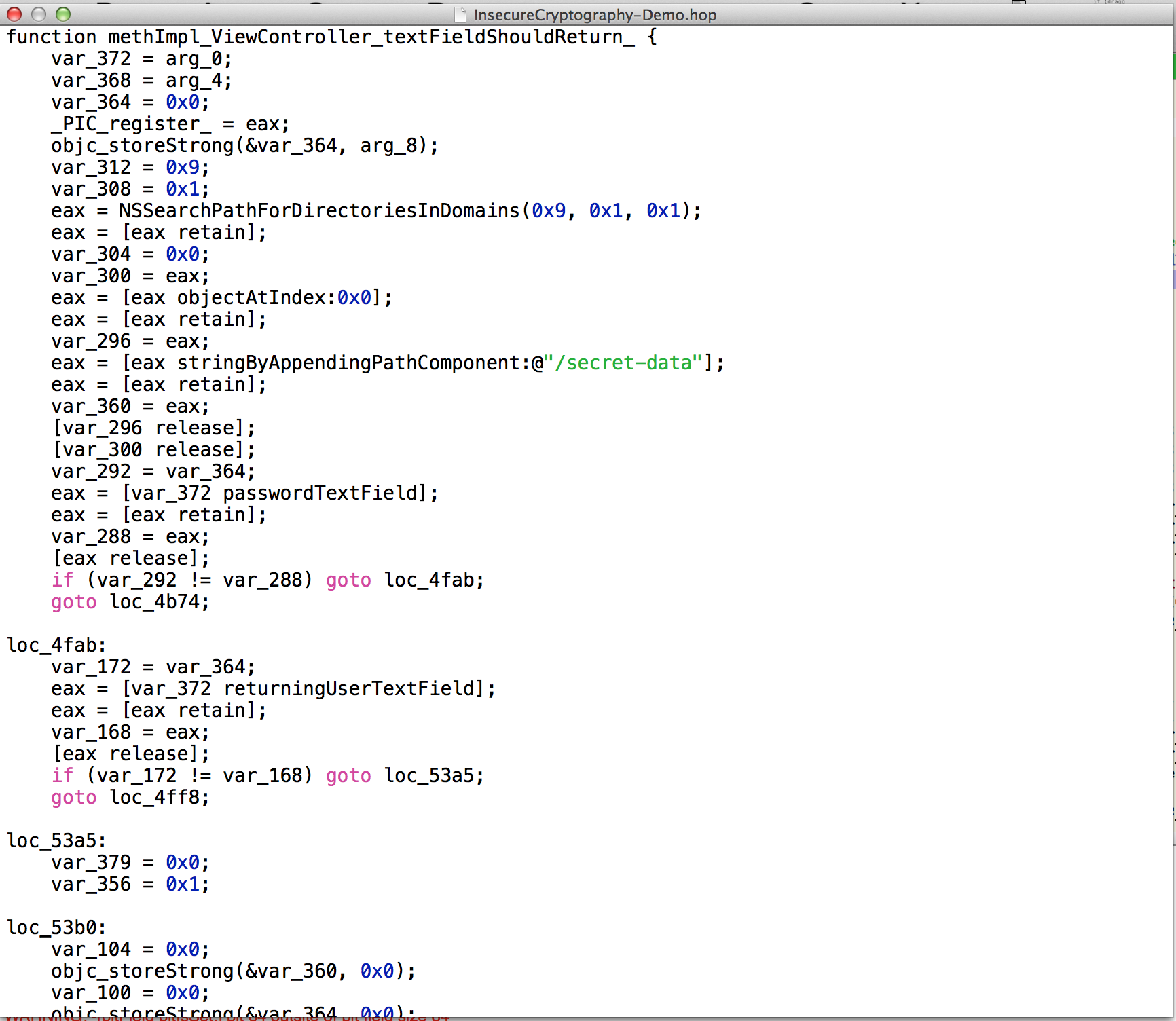Well, this pretty much gives everything away. And this is why i love Hopper so much. Here is the pseudo code generated for this method.

```    function methImpl_ViewController_textFieldShouldReturn_ {
var_372 = arg_0;
var_368 = arg_4;
var_364 = 0x0;
_PIC_register_ = eax;
objc_storeStrong(&var_364, arg_8);
var_312 = 0x9;
var_308 = 0x1;
eax = NSSearchPathForDirectoriesInDomains(0x9, 0x1, 0x1);
eax = [eax retain];
var_304 = 0x0;
var_300 = eax;
eax = [eax objectAtIndex:0x0];
eax = [eax retain];
var_296 = eax;
eax = [eax stringByAppendingPathComponent:@"/secret-data"];
eax = [eax retain];
var_360 = eax;
[var_296 release];
[var_300 release];
var_292 = var_364;
eax = [eax retain];
var_288 = eax;
[eax release];
if (var_292 != var_288) goto loc_4fab;
goto loc_4b74;

loc_4fab:
var_172 = var_364;
eax = [var_372 returningUserTextField];
eax = [eax retain];
var_168 = eax;
[eax release];
if (var_172 != var_168) goto loc_53a5;
goto loc_4ff8;

loc_53a5:
var_379 = 0x0;
var_356 = 0x1;

loc_53b0:
var_104 = 0x0;
objc_storeStrong(&var_360, 0x0);
var_100 = 0x0;
objc_storeStrong(&var_364, 0x0);
eax = SIGN_EXTEND(var_379);
return eax;

loc_4ff8:
eax = [var_372 returningUserTextField];
eax = [eax retain];
var_164 = eax;
eax = [eax text];
eax = [eax retain];
var_160 = 0x4;
var_156 = eax;
eax = [eax dataUsingEncoding:0x4];
eax = [eax retain];
var_336 = eax;
[var_156 release];
[var_164 release];
var_332 = 0x0;
eax = [NSData dataWithContentsOfFile:var_360];
eax = [eax retain];
var_328 = eax;
var_320 = var_332;
eax = [RNDecryptor decryptData:var_328 withPassword:@"Secret-Key" error:&var_320];
eax = [eax retain];
var_152 = eax;
objc_storeStrong(&var_332, var_320);
var_324 = var_152;
eax = [var_336 isEqualToData:var_324];
if (eax != 0x0) {
eax = [var_372 loggedInLabel];
eax = [eax retain];
var_148 = 0x0;
var_144 = eax;
[eax setHidden:0x0];
[var_144 release];
eax = [var_372 returningUserTextField];
eax = [eax retain];
var_140 = 0x1;
var_136 = eax;
[eax setHidden:0x1];
[var_136 release];
eax = [var_372 returningUserLabel];
eax = [eax retain];
var_132 = 0x1;
var_128 = eax;
[eax setHidden:0x1];
[var_128 release];
var_356 = 0x0;
}
else {
var_124 = @"OK";
var_120 = @"Oops";
var_112 = 0x0;
eax = [eax initWithTitle:var_120 message:var_116 delegate:0x0 cancelButtonTitle:var_124 otherButtonTitles:0x0];
var_108 = eax;
[eax show];
[var_108 release];
var_379 = 0x0;
var_356 = 0x1;
}
ecx = esp;
*ecx = &var_324;
*(ecx + 0x4) = 0x0;
objc_storeStrong();
ecx = esp;
*ecx = &var_328;
*(ecx + 0x4) = 0x0;
objc_storeStrong();
ecx = esp;
*ecx = &var_332;
*(ecx + 0x4) = 0x0;
objc_storeStrong();
ecx = esp;
*ecx = &var_336;
*(ecx + 0x4) = 0x0;
objc_storeStrong();
if (var_356 != 0x0) goto loc_53b0;
goto loc_53a5;

loc_4b74:
eax = [var_364 resignFirstResponder];
var_287 = eax;
eax = [var_364 text];
eax = [eax retain];
var_280 = eax;
eax = [eax length];
var_276 = eax;
[var_280 release];
if (var_276 != 0x0) goto loc_4c9b;
goto loc_4be5;

loc_4c9b:
eax = [eax retain];
var_252 = eax;
eax = [eax text];
eax = [eax retain];
var_248 = 0x4;
var_244 = eax;
eax = [eax dataUsingEncoding:0x4];
eax = [eax retain];
var_352 = eax;
[var_244 release];
[var_252 release];
var_348 = 0x0;
var_340 = var_348;
edi = esp;
var_240 = 0x12;
var_236 = _kRNCryptorAES256Settings;
var_232 = *0x158b0;
var_228 = edi;
var_224 = *0x157bc;
*(edi + 0xc) = *var_236;
esi = var_228;
*(esi + 0x58) = &var_340;
*(esi + 0x54) = @"Secret-Key";
*(esi + 0x8) = var_352;
*(esi + 0x4) = var_224;
*esi = var_232;
eax = objc_msgSend();
eax = [eax retain];
var_220 = eax;
objc_storeStrong(&var_348, var_340);
var_344 = var_220;
var_216 = 0x1;
eax = [var_344 writeToFile:var_360 atomically:0x1];
var_215 = eax;
eax = [NSUserDefaults standardUserDefaults];
eax = [eax retain];
var_208 = eax;
var_204 = 0x1;
[eax setBool:0x1 forKey:@"loggedIn"];
[var_208 release];
eax = [NSUserDefaults standardUserDefaults];
eax = [eax retain];
var_200 = eax;
eax = [eax synchronize];
var_199 = eax;
[var_200 release];
eax = [var_372 firstUserView];
eax = [eax retain];
var_192 = 0x1;
var_188 = eax;
[eax setHidden:0x1];
[var_188 release];
var_184 = 0x0;
objc_storeStrong(&var_344, 0x0);
var_180 = 0x0;
objc_storeStrong(&var_348, 0x0);
var_176 = 0x0;
objc_storeStrong(&var_352, 0x0);
goto loc_53a5;

loc_4be5:
var_272 = @"OK";
var_268 = @"Oops";
var_260 = 0x0;
eax = [eax initWithTitle:var_268 message:var_264 delegate:0x0 cancelButtonTitle:var_272 otherButtonTitles:0x0];
var_256 = eax;
[eax show];
[var_256 release];
var_379 = 0x0;
var_356 = 0x1;
goto loc_53b0;
}

```

Some things that we can interpret from this Pseudo code.

1. ```eax = [eax text];
eax = [eax retain];
var_160 = 0x4;
var_156 = eax;
eax = [eax dataUsingEncoding:0x4];```

The text from the text field is being converted into NSData using the method dataUsingEncoding.

2. ```eax = NSSearchPathForDirectoriesInDomains(0x9, 0x1, 0x1);
eax = [eax retain];
var_304 = 0x0;
var_300 = eax;
eax = [eax objectAtIndex:0x0];
eax = [eax retain];
var_296 = eax;
eax = [eax stringByAppendingPathComponent:@"/secret-data"];```

This indicates a file with the name secret-data. If we scroll down a bit, we see this line

` eax = [var_344 writeToFile:var_360 atomically:0x1];`

Looks like something is being written to this file.

3. ```        var_332 = 0x0;
eax = [NSData dataWithContentsOfFile:var_360];
eax = [eax retain];
var_328 = eax;
var_320 = var_332;
eax = [RNDecryptor decryptData:var_328 withPassword:@"Secret-Key" error:&var_320];
```

The contents of the file with the name Secret-data is being read and decrypted with a password.

4. `eax = [RNDecryptor decryptData:var_328 withPassword:@"Secret-Key" error:&var_320];`

It looks like the key used for enryption and decryption is a hardcoded string Secret-Key.

5. `eax = [var_336 isEqualToData:var_324];`

There is a comparison between two kinds of data. The boolean value result is stored in the eax register.

6. ```if (eax != 0x0) {
eax = [var_372 loggedInLabel];
eax = [eax retain];
var_148 = 0x0;
var_144 = eax;
[eax setHidden:0x0];
[var_144 release];
eax = [var_372 returningUserTextField];
eax = [eax retain];
var_140 = 0x1;
var_136 = eax;
[eax setHidden:0x1];
[var_136 release];
eax = [var_372 returningUserLabel];
eax = [eax retain];
var_132 = 0x1;
var_128 = eax;
[eax setHidden:0x1];
[var_128 release];
var_356 = 0x0;
}
else {
var_124 = @"OK";
var_120 = @"Oops";
var_112 = 0x0;
eax = [eax initWithTitle:var_120 message:var_116 delegate:0x0 cancelButtonTitle:var_124 otherButtonTitles:0x0];
var_108 = eax;
[eax show];
[var_108 release];
var_379 = 0x0;
var_356 = 0x1;
}```

Depending on the value of eax, the flow can go to different places. If the value is 0, user will be shown an alert that the password is incorrect. Otherwise, as you might be guessing, the user is logged in.

Well, lets see if we can find the file secret-data in the application sandbox. On searching just a little bit, we see that this file is present in the Documents folder. On opening it, we find that it contains some data. and the content looks encrypted.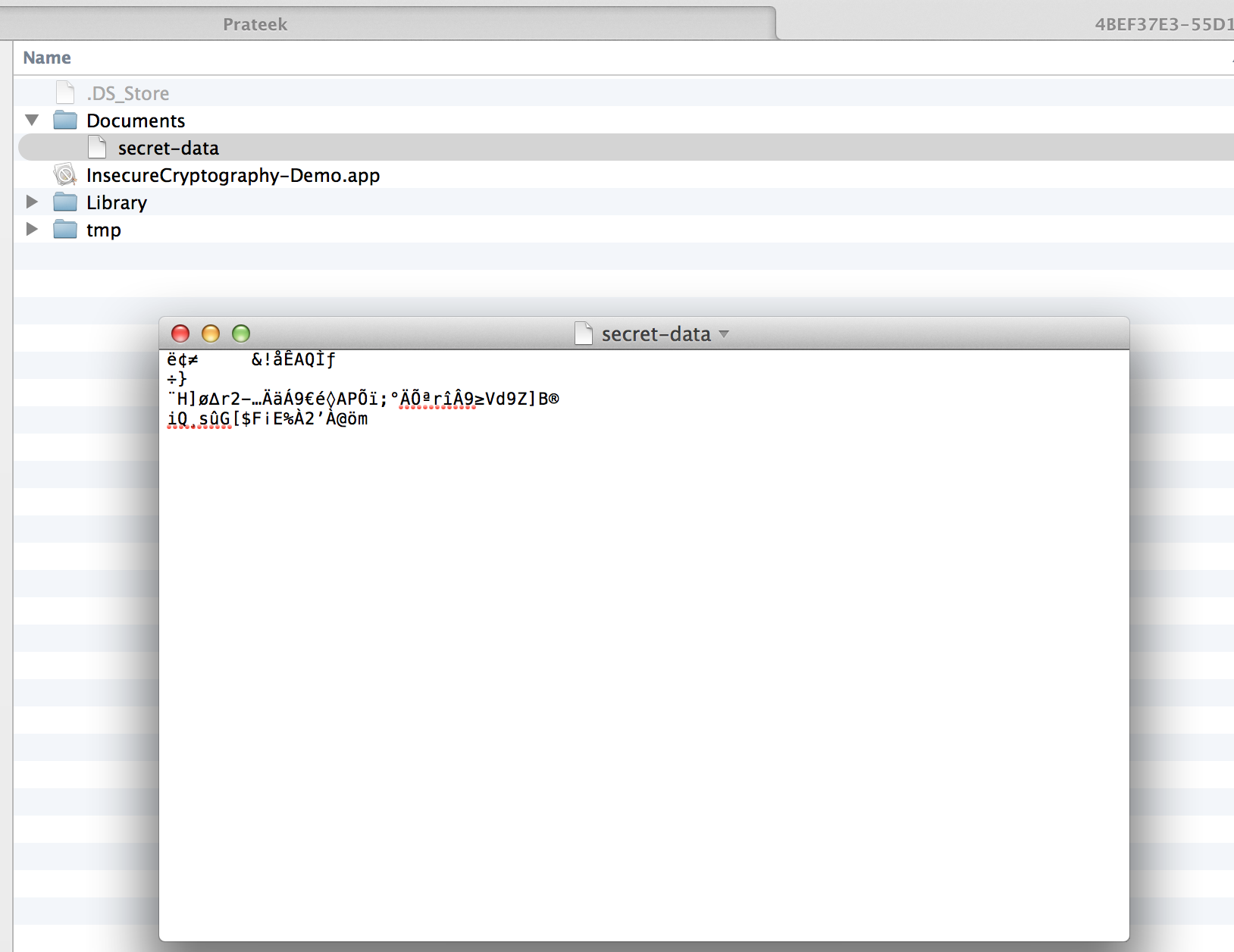Also, on googling a bit on RNEncryptor and RNDecryptor, we see that they are part of an open source library available on Github that can be found here

So from all this information, we can interpret that.

• When the user enters the password, the text is converted into NSData using the method dataUsingEncoding with the param 0x4. The parameter 0x4 corresponds to NSUTF8StringEncoding.
• Data is read from the file secret-data and decrypted using a hardcoded key
• The two values found from the above two steps are compared against each other. If they match, the user is logged in.

Well, it is pretty much clear that we can find the password by decrypting the data from the file secret-data and converting it into a string with the encoding NSUTF8StringEncoding. Let’s write a simple iOS Application to decrypt the data. For this, you will need to copy the file secret-data from the application sandbox and paste it into the documents folder of the application’s sandbox of this new application. You can also download the complete code from here.

We add this method in the new project.

```NSString *dataPath = [[NSSearchPathForDirectoriesInDomains(NSDocumentDirectory, NSUserDomainMask, YES) objectAtIndex:0] stringByAppendingPathComponent:@"/secret-data"];
NSError *error;
NSData *encryptedData = [NSData dataWithContentsOfFile:dataPath];
NSData *decryptedData = [RNDecryptor decryptData:encryptedData
error:&error];
NSString *password = [[NSString alloc] initWithData:decryptedData
encoding:NSUTF8StringEncoding];

UILabel *newLabel = [[UILabel alloc] initWithFrame:CGRectMake(140.0, 160.0, 100.0, 100.0)];
```

As you can clearly note, this method decrypts the data using the hardcoded password, and shows the value in a label that is displayed on the view.

After running this application, we can easily see the decrypted password.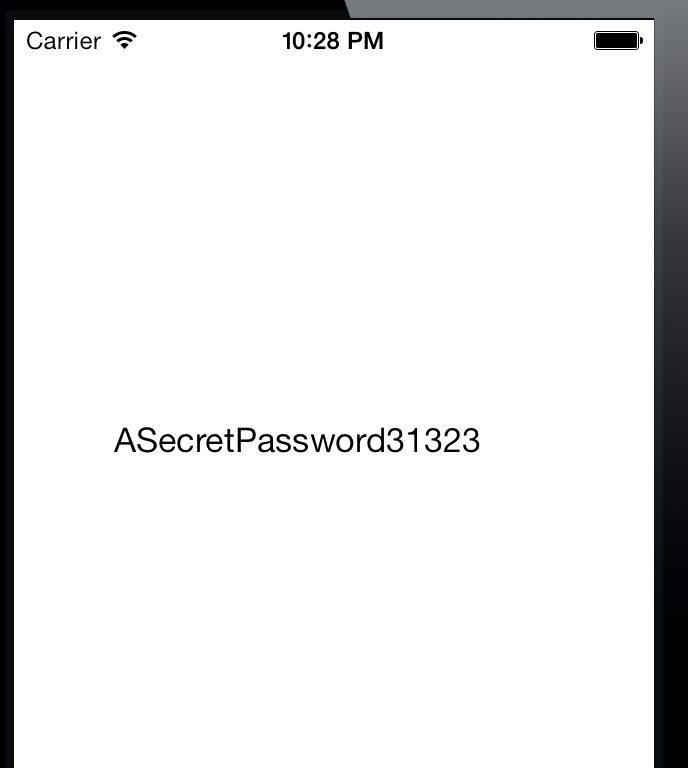In this article, we looked at how one can exploit a weakness in the encryption being used to find sensitive information from an application. In this case, the weakness was using a hardcoded key. It is essential for developers to make sure that they enforce proper encryption in their applications to prevent them from being compromised.

In the next article, we will look at Client Side Injection in iOS Applications.

*